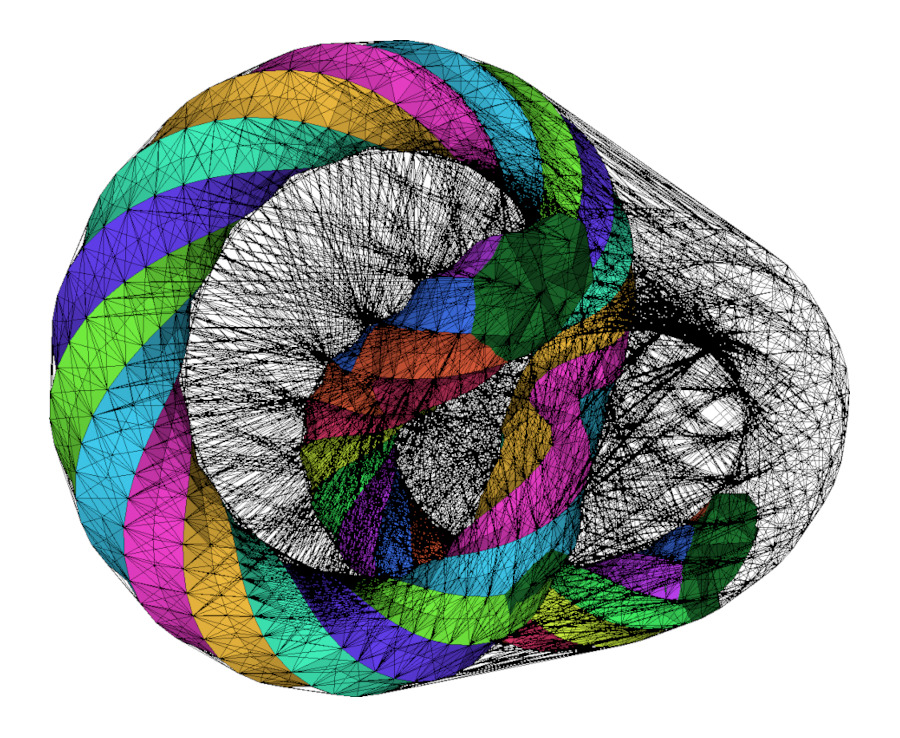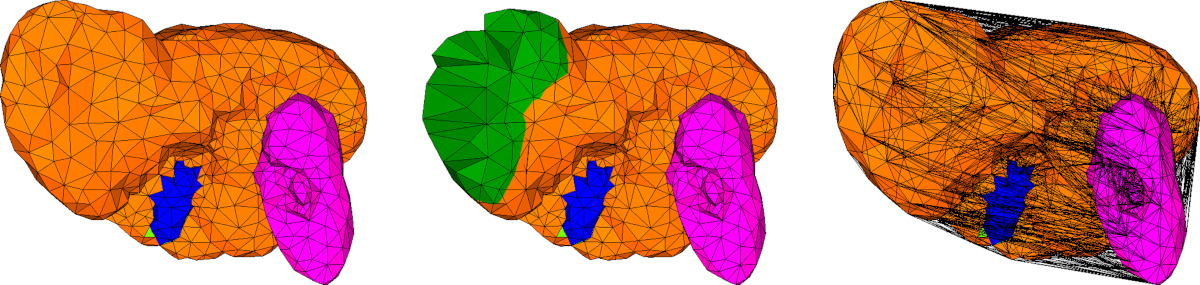CGAL 5.6 - 3D Simplicial Mesh Data Structures
User ManualFigure 58.1 A multi-domain tetrahedral mesh generated from a polyhedral surface with multiple surface patches. The complete triangulation is displayed, including cells that belong or do not belong to the mesh complex, surface patches, and feature edges.

# Mesh Complex

This package is devoted to the representation of 3-Dimensional simplicial mesh data structures.

A 3D simplicial complex is composed of points, line segments, triangles, tetrahedra, and their corresponding combinatorial description (namely vertices, edges, faces and cells). CGAL provides 3D triangulations, that describe both the geometry and connectivity of a 3D simplicial complex, implemented in the the 3D Triangulations and 3D Triangulation Data Structure packages.

We introduce the concept of mesh complex, that encodes extra information on top of a 3D triangulation to represent a valid simplicial complex. A mesh complex describes four subcomplexes of simplices of the support 3D triangulation, per dimension from 0 to 3:

• corner vertices (0D),
• feature edges (1D),
• surface facets (2D),
• domain cells (3D). See Figure Figure 58.2.

The concept MeshComplex_3InTriangulation_3 is a data structure devised to represent these three-dimensional complexes embedded in a Triangulation_3.Figure 58.2 (Left and Middle) A multi-domain 3D mesh is represented by its tetrahedral cells, with subdomain indices, and surface indices (depicted with different colors here). (Right) The underlying Triangulation_3 triangulates the whole convex hull of its vertices. The cells that lie outside the meshing domain are drawn in wire frame.

# Examples

## From Tetrahedron Soup to Triangulation_3

In the first example of this section, we build a random Delaunay_triangulation_3 and use it to build a consistent though connectivity-free tetrahedron soup. The tetrahedron soup is then put back together in a CGAL::Tetrahedral_remeshing::Remeshing_triangulation_3 before being set as the reference triangulation of a Mesh_complex_3_in_triangulation_3.

#include <CGAL/Exact_predicates_inexact_constructions_kernel.h>
#include <CGAL/Delaunay_triangulation_3.h>
#include <CGAL/point_generators_3.h>
#include <CGAL/Tetrahedral_remeshing/Remeshing_triangulation_3.h>
#include <CGAL/Mesh_complex_3_in_triangulation_3.h>
#include <CGAL/tetrahedron_soup_to_triangulation_3.h>
#include <CGAL/IO/File_medit.h>
#include <vector>
#include <unordered_map>
using Point_3 = K::Point_3;
using Tetrahedron_3 = K::Tetrahedron_3;
using Vertex_handle = DT3::Vertex_handle;
using Subdomain_index = C3T3::Subdomain_index;
int main(int , char* [])
{
const int nbv = 100;
//a triangulation
DT3 delaunay;
std::unordered_map<Vertex_handle, int> v2i;
std::vector<DT3::Point> points(nbv);
std::vector<Tetrahedron_3> tetrahedra;
std::vector<std::array<int, 4> > tets_by_indices;
std::vector< Subdomain_index> subdomains;
//insert random points
CGAL::Random_points_in_cube_3<Point_3> randp(2.);
int i = 0;
while (i < nbv)
{
points[i] = *randp++;
Vertex_handle v = delaunay.insert(points[i]);
v2i[v] = i++;
}
tetrahedra.reserve(delaunay.number_of_finite_cells());
tets_by_indices.reserve(delaunay.number_of_finite_cells());
subdomains.reserve(delaunay.number_of_finite_cells());
for (DT3::Cell_handle c : delaunay.finite_cell_handles())
{
tetrahedra.push_back(delaunay.tetrahedron(c));
tets_by_indices.push_back( { v2i.at(c->vertex(0)),
v2i.at(c->vertex(1)),
v2i.at(c->vertex(2)),
v2i.at(c->vertex(3)) } );
subdomains.push_back(Subdomain_index(1));
}
//build triangulation from tetrahedra
Remeshing_triangulation tr
= CGAL::tetrahedron_soup_to_triangulation_3<Remeshing_triangulation>(tetrahedra);
//build triangulation from indices
Remeshing_triangulation tr2
= CGAL::tetrahedron_soup_to_triangulation_3<Remeshing_triangulation>(
points, tets_by_indices,
CGAL::parameters::subdomain_indices(std::cref(subdomains)));
//build a C3T3
C3T3 c3t3;
c3t3.triangulation() = tr;
std::ofstream ofs("c3t3_output.mesh");
return EXIT_SUCCESS;
}

## Input/Output Example

The example below illustrates how to use the IO functions for reading and writing a triangulation with the Medit file format (See  for a comprehensive description of this file format.).

#include <CGAL/Exact_predicates_inexact_constructions_kernel.h>
#include <CGAL/Triangulation_3.h>
#include <CGAL/Triangulation_data_structure_3.h>
#include <CGAL/Simplicial_mesh_cell_base_3.h>
#include <CGAL/Simplicial_mesh_vertex_base_3.h>
#include <CGAL/Mesh_complex_3_in_triangulation_3.h>
#include <CGAL/tetrahedral_remeshing.h>
#include <CGAL/tags.h>
#include <CGAL/IO/File_medit.h>
#include <fstream>
using Subdomain_index = int;
using Surface_patch_index = unsigned char;
using Curve_index = char;
using Corner_index = short;
using Vb = CGAL::Simplicial_mesh_vertex_base_3<K, Subdomain_index, Surface_patch_index,
Curve_index, Corner_index>;
using Triangulation = CGAL::Triangulation_3<K, Tds>;
int main(int argc, char* argv[])
{
std::cout.precision(17);
std::cerr.precision(17);
std::string filename = (argc > 1) ? std::string(argv)
: CGAL::data_file_path("meshes/elephant.mesh");
Triangulation tr;
std::ifstream is(filename, std::ios_base::in);
{
std::cerr << "Failed to read" << std::endl;
return EXIT_FAILURE;
}
// [call a remeshing algorithm]
std::ofstream os("after_remeshing.mesh");
CGAL::IO::write_MEDIT(os, tr, CGAL::parameters::all_vertices(true));
os.close();
Triangulation tr2;
std::ifstream is2("after_remeshing.mesh");
{
std::cerr << "Failed to read (#2)" << std::endl;
return EXIT_FAILURE;
}
std::cout << "Done" << std::endl;
return EXIT_SUCCESS;
}

## More Examples In Other Packages

The Mesh_complex_3_in_triangulation_3 is widely used in the 3D Mesh Generation package. Many more examples can be found in its Examples section.

The package Tetrahedral Remeshing also makes use of the Mesh_complex_3_in_triangulation_3 since it serves as a post-processing for tetrahedral mesh generation. Some examples can be found in the Examples section.

# Implementation History

The code of the MeshComplex_3InTriangulation_3 and its variants were initially part of the package 3D Mesh Generation. With the meshing and remeshing processes becoming more versatile, it was moved to its own package in the release 5.6 of CGAL.